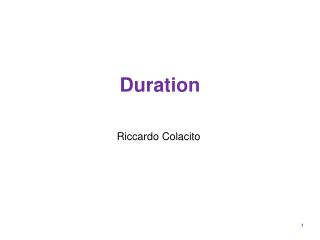DownloadDownload PresentationDuration

# Duration

Download Presentation## Duration

- - - - - - - - - - - - - - - - - - - - - - - - - - - E N D - - - - - - - - - - - - - - - - - - - - - - - - - - -
##### Presentation Transcript

1. Duration Riccardo Colacito

2. Motivational example • Two bonds sell today with the same payment schedule • Time 1: \$10 • Time 2: \$20 • However one has a YTM of 1% and the other one has a YTM of 99% • What is the temporal distribution of the payments?

3. Example (cont’d)

4. Effective maturity? • Both bonds have maturity 2 years • But they differ in the temporal distribution of the (present value of) payments • Their effective maturities should differ, i.e. the second one should have shorter maturity

5. Duration • A measure of the effective maturity of a bond • The weighted average of the times until each payment is received, with the weights proportional to the present value of the payment • Duration is always shorter or equal to maturity for all bonds

6. Duration is where Duration: Calculation

7. Duration: an example • Consider the following bond • Maturity: 4 years • Par: \$500 • Coupon: \$80 (once per year) • YTM: 38.5% • Price is \$287.205 (remember how to compute this?)

8. Compute the duration

9. Just use the formula … or three years Duration is…

10. Cash flows and their present values

11. Question • What happens if the YTM decreases? • Would you expect the duration to increase or decrease? • Why?

12. Duration: another example • Consider the following bond • Maturity: 4 years • Par: \$500 • Coupon: \$80 (once per year) • YTM: 15.6% 38.5% • Price is \$505.641 \$287.205

13. How are the weights affected?

14. What happens to the duration? • You now attach a relatively higher weight to cash flows that happen further in the future • That is: the duration goes up! • Can verify that the duration is now 3 years and a quarter.

15. Question • What is the duration of a zero coupon bond? • The duration of a zero coupon bond is equal to its maturity

16. A perpetuity • Definition: a security that pays a constant coupon forever • Its maturity is infinite! • Its duration is

17. Duration vs maturity • Holding the coupon rate constant, a bond’s duration generally increases with time to maturity

18. Duration vs maturity

19. Duration vs Maturity (cont’d)

20. Why does this happen? • As the YTM increases, the present value of the face value at high maturities gets smaller and smaller. • That is the bond starts looking as a perpetuity with a very high yield to maturity.

21. Figure 10.3 Duration as a Function of Maturity

22. Uses of Duration • Summary measure of length or effective maturity for a portfolio • Measure of price sensitivity for changes in interest rate • Immunization of interest rate risk (next class)

23. Duration/Price Relationship • Take derivative of price wrt YTM • Hence the following holds as an approximation

24. More compact formula where Modified Duration

25. Fact #1 • Prices of long-term bonds are more sensitive to interest rate changes than prices of short term bonds

26. Fact #1

27. Intutition • The higher the maturity, the higher the duration • The higher the duration, the higher the price sensitivity • Higher maturity implies higher price sensitivity to interest rate risk

28. Fact #2 • The sensitivity of bond prices to changes in yields increases at a decreasing rate as maturity increases. • Equivalently, interest rate risk is less than proportional to bond maturity.

29. Fact #2

30. Intuition • Duration increases less than proportionally with maturity (e.g. see slide 18) • Hence price sensitivity to interest rate risk increases less than proportionally with maturity.

31. Fact #3 • Prices of high coupon bonds are less sensitive to changes in interest rates than prices of low coupon bonds. • Or equivalently, interest rate risk is inversely related to the bond’s coupon rate

32. Fact #3

33. Intuition • The higher the coupon, the lower the duration • Lower duration implies lower price sensitivity. • The higher the coupon, the lower the price sensitivity to interest rate risk

34. Fact #4 • The sensitivity of a bond’s price to a change in its yield is inversely related to the yield to maturity at which the bond currently is selling.

35. Fact #4

36. Intuition • The higher the YTM, the lower the duration • The lower the duration, the lower the price sensitivity • High YTM implies low price sensitivity

37. Convexity • The formula is an approximation of the more precise relation

38. Figure 10.6 Bond Price Convexity

39. The higher the convexity, the better it is for the investor

40. Yet another fact • An increase in a bond’s yield to maturity results in a smaller price change than a decrease in yield of equal magnitude